# What is Ohm's law in vector form.........???

@sunil.sahay92 has answered this question very well. Keep it up. This has been a great help to the users of this community. However I will briefly explain the Ohm’s law in vector form below.

Simply ohm’s law is:

V = IR where V is the potential difference, I is the current and R is the resistance.

Also R = pl/A where p is the resistivity which is material dependent parameter.

Now current density J is a vector quantity given by Jz = I/A if the conductor is aligned along z axis.

Substituting R in V = IR,

V = I * pl/A

Putting value of I/A = J

V = plJ

Also V/l is E which is a vector quantity , so

E = pJ

Or J = σE

Here σ is called conductivity and is 1/p , it is also material dependent.

• 55

simplest version of Ohm's law: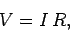whereis the voltage drop across a resistor of resistancewhen a current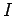flows through it. Let us generalize this law so that it is expressed in terms of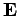and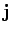, rather thanand. Consider a length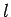of a conductor of uniform cross-sectional areawith a currentflowing down it. In general, we expect the electrical resistance of the conductor to be proportional to its length, and inversely proportional to its area (i.e., it is harder to push an electrical current down a long rather than a short wire, and it is easier to push a current down a wide rather than a narrow conducting channel.) Thus, we can write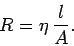The constant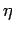is called the resistivity, and is measured in units of ohm-meters. Ohm's law becomes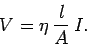However,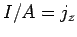(supposing that the conductor is aligned along the-axis) and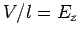, so the above equation reduces to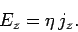There is nothing special about the-axis (in an isotropic conducting medium), so the previous formula immediately generalizes to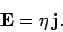This is the vector form of Ohm's law.

• 5

thank u vry vry much.......:)...♣

• -18
What are you looking for?